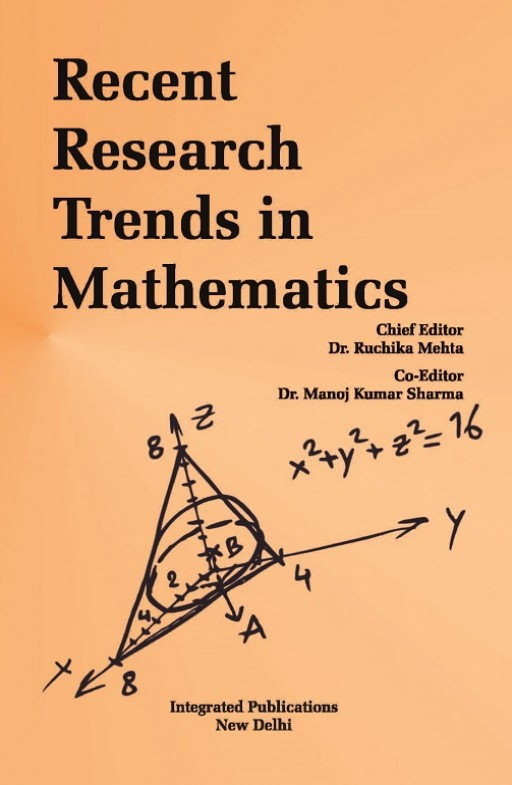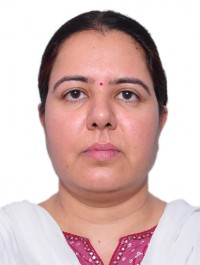# Publish a Book Chapter in "Recent Research Trends in Mathematics (Volume - 5)"

## Mathematics Edited Book | Edited Book on Mathematics

This edited book on mathematics titled "Recent Research Trends in Mathematics" mainly focuses on various topics such as algebra, linear and multilinear algebra; matrix theory etc., and the rest are given below in the Scope of the book. This mathematics edited book will be published with ISBN and DOI numbers after following a proper double blind peer reviewed process. All the chapters of this mathematics edited book will be published in a very illustrative manner for easy reader understanding.Book Scope

• Algebra
• Linear and Multilinear algebra; matrix theory
• Geometry
• Real functions
• Measure and integration
• Functions of a complex variable
• Several complex variables and analytic spaces
• Ordinary differential equations
• Partial differential equations
• Dynamical systems and ergodic theory
• Difference and functional equations
• Fourier analysis
• Integral equations
• Functional analysis
• Operator theory
• Calculus of variations and optimal
• control; optimization
• Operations research
• mathematical programming
• Differential geometry
• General topology
• Manifolds and cell complexes
• Global analysis
• analysis on manifolds
• Probability theory and stochastic processes
• Statistics
• Numerical analysis
• Computer science
• Mechanics of particles and systems
• Fluid mechanics
• Mechanics of deformable solids
• electromagnetic theory
• Optics
• Quantum theory
• Statistical mechanics

Author Guidelines

### Chief EditorDr. Ruchika Mehta

Associate Professor, Department of Mathematics & Statistics, Manipal University, Jaipur, Rajasthan, India

Coeditor(s)Dr. Manoj Kumar Sharma

Associate Professor, Manipal University, Jaipur, IndiaDr. Savita Deswal

Associate Professor, Department of Mathematics, Govt. P.G. College for Women, Rohtak, Haryana, India

How To Submit

Email your book chapter at printintegrated@gmail.com

Customer helpline number +91-9953533410

31 Dec 2023

Submit Chapter